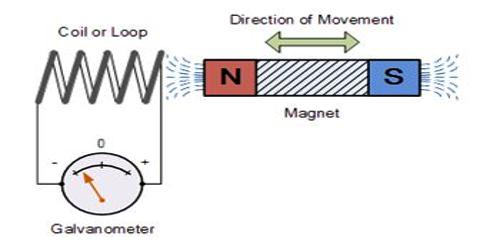# Significance of the Faraday’s Laws

Significance of the Faraday’s Laws

Faraday’s law of induction is one of the important concepts of electricity. It looks at the way changing magnetic fields can cause current to flow in wires. Using the first law it was found that the quantity of electricity required to deposit one mole of silver, was 96,500 Coulombs. Now one Ion of silver is deposited at the cathode as an atom of silver as follows:

Ag+ + e = Ag

That is to say that one electron deposits one atom of silver. To deposit one mole of silver one mole of electrons will be required. Hence one mole of electrons carries 96,500 Coulombs of electricity. In honour of Michael Faraday 96,500 Coulombs of electricity was named as one Faraday of electricity. So we write,

I F = 96,500 Coulombs = 96,500 C (C stands for Coulomb)

and we say that to deposit one mole of solver 1 F of electricity are required.

Now according to the First law of Faraday, 1 f will deposit one mole of silver, 2 F will deposit 2 moles of Ag and 3 F will deposit 3 moles of silver.

Since one mole of electron carry 96,500 Coulombs of electricity the charge carried by one electron = 996,500 / 6.02 x 1027) C = 1.60 x 10-19 C.

Copper and aluminum are deposited as follows:

Ca2+ + 2e = Cu

Al3+ + 3e = Al

It follows that to deposit one mole of copper two moles of electrons are required, i.e., 2 F of electricity we required and to deposit one mole of Aluminum 3 F are required. When 96,500 C or 1 F of electricity is passed through separate electrolytic cells containing Ag+, Cu2+ and Al3+ ions respectively the amount of metal deposited at the cathodes in the different cells were found to be Ag, 1 mol, Cu, ½ mol, Al, 1/3 mol i.e., in each case the  amount of metal deposited

= [1.0 mol / number of changes of the ion]

As mentioned earlier these amounts are what Faraday referred to as the equivalent masses of the metals in the second law.

We will see later that to discharge one mole of oxygen at the anode the reaction is –

4 OH = 2 H2O + O2 + 4e

and 4F of electricity will be required.